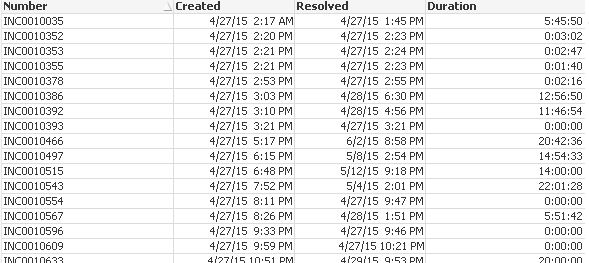# New to QlikView

Discussion board where members can get started with QlikView.

Announcements
Leverage your QlikView investment to modernize BI – see how! Join Group
cancel
Showing results for
Did you mean:
HighlightedNot applicable

## % Less than 3 days

Hello,

I need to get the % of tickets closed in 3 days or less.  I tried the formula below but it isn't working and I am not really sure how to do this.

=count(if(Duration>=30,Number)/Count(Number)

Here is my data:Duration is the amount of time the ticket was open.  So I need to count the number of incidents that were open less than 36 hours and divide that by the number of total incidents.

Any help would be much appreciated.

1 Solution

Accepted Solutions
HighlightedMVP

## Re: % Less than 3 days

this is for duration less or equal 3 days

=count({\$ <Duration={"<=\$(=Interval(3))"}>} Number) / count(Number)

3 Replies
HighlightedMaster

## Re: % Less than 3 days

Hi Jason,

in script

If(Len(Trim(Resolved)) = 0

If((interval(Date(Resolved,'DD-MM-YYYY hh:mm:ss')-Date(Created,'DD-MM-YYYY hh:mm:ss'),'hh'))<=36,'Ópen','Closed') as OpenByAge.

Use OpenByAge flag in ui:

=count(if(OpenByAge = 'Ópen',Number)/Count(Number)

Regards

Neetha

HighlightedMVP

## Re: % Less than 3 days

=count({\$ <Duration={">=\$(=Interval(1.5))"}>} Number) / count(Number)

see attachment

HighlightedMVP

## Re: % Less than 3 days

this is for duration less or equal 3 days

=count({\$ <Duration={"<=\$(=Interval(3))"}>} Number) / count(Number)